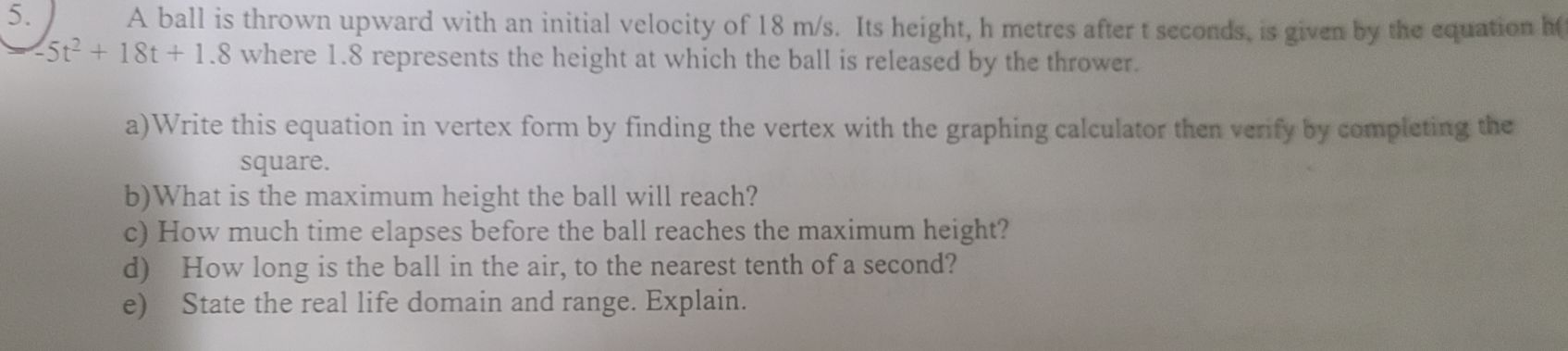### Still have math questions?

Algebra
QuestionA ball is thrown upward with an initial velocity of $$18 m / s$$ . Its height, h metres after $$t$$ seconds, is given by the equation he $$- 5 t ^ { 2 } + 18 t + 1.8$$ where $$1.8$$ represents the height at which the ball is released by the thrower.

a) Write this equation in vertex form by finding the vertex with the graphing calculator then verify by completing the square.

b) What is the maximum height the ball will reach?

c) How much time elapses before the ball reaches the maximum height?

d) How long is the ball in the air, to the nearest tenth of a second?

e) State the real life domain and range. Explain.

(a) Vertex is at $$( 1.8,18)$$

(b) The maximum height the ball will reach is the y component of the vertex = 18 m

(c) The time that elapses before ball reaches maximum height is the x component of the vertex = 1.8 s

(d) This it the difference in time from the point of release (y-intercept) and the point at the which the ball fell (second root)

Time of flight = 3.7 - 0 = 3.7 s

(e) Real life domain is $$[ 0,3.7]$$

This it the time it took the ball to be released (y-intercept) and hit the ground (second root)

Solution
View full explanation on CameraMath App.